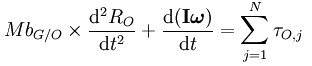# Can someone explain this can't understand notation

carrotstien
from wikipedia for
"The most general equation for rotation of a rigid body in three dimensions about an arbitrary origin O with axes x, y, z is"...i thought that the sum of the torques on a system is just equal to d/dt(L)=d/dt(Iw)...apparently not - but i can't understand the notation like, what is b, G..etc

## Answers and Replies

Gold Member
http://www.statemaster.com/encyclopedia/Rigid-body-dynamics

I is the moment of inertia tensor
ω is the angular velocity (a vector)
ωq is the angular velocity about axis q.
M is the total mass.
bG/O is the vector from O to the body's center of mass.
RO is the position of O.
t is time.
τO,j is one of the N moments about O.